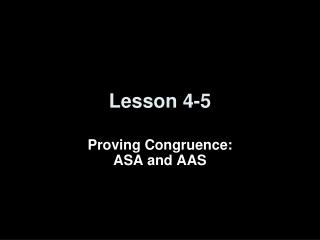DownloadDownload PresentationLesson 4-5

# Lesson 4-5

Télécharger la présentation## Lesson 4-5

- - - - - - - - - - - - - - - - - - - - - - - - - - - E N D - - - - - - - - - - - - - - - - - - - - - - - - - - -
##### Presentation Transcript

1. Lesson 4-5 Proving Congruence:ASA and AAS

2. Transparency 4-5 5-Minute Check on Lesson 4-4 Determine which postulate can be used to prove that the triangles are congruent. If it is not possible to prove they are congruent, write not possible. 1. 2. 3. 4. 5. 6. If AB RS and BC  ST, what additional congruence statement would be necessary to proveABCRST by the SAS postulate? Standardized Test Practice: BS D C AT A AR B CT

3. Transparency 4-5 5-Minute Check on Lesson 4-4 Determine which postulate can be used to prove that the triangles are congruent. If it is not possible to prove they are congruent, write not possible. 1. 2. 3. SAS SSS SSS 4. 5. not possible SSS 6. If AB RS and BC  ST, what additional congruence statement would be necessary to proveABCRST by the SAS postulate? Standardized Test Practice: BS D C AT A AR B CT

4. Objectives • Use the ASA Postulate to test for triangle congruence • Use the AAS Theorem to test for triangle congruence

5. Vocabulary • Included side – the side in common between two angles (end points are the vertexes)

6. Postulates and Theorems • Angle-Side-Angle (ASA) Postulate:If two angles and the included side of one triangle are congruent to two angles and the included side of another triangle, then the triangles are congruent. • Angle-Angle-Side (AAS) Theorem:If two angles and a non-included side of one triangle are congruent to the corresponding two angles and side of another triangle, then the triangles are congruent.

7. Given:AC = CD AD Prove:ABCDEC Reasons Statements ADGiven in problem AC = CD (included side)Given ACBDCE ABCDEC Angle – Side – Angle (ASA) Vertical Angles Theorem ASA Postulate

8. Write a paragraph proof. Given:L is the midpoint of Prove:WRL EDL Proof: because alternate interior angles are congruent. By the Midpoint Theorem, Since vertical angles are congruent, WRL EDLby ASA.

9. Given: Prove: Write a flow proof. Proof:

10. Write a flow proof. Given: Prove: Proof:

11. STANCES When Ms. Gomez puts her hands on her hips, she forms two triangles with her upper body and arms. Suppose her arm lengths AB and DE measure 9 inches, andAC and EF measure 11 inches. Also suppose thatyou are given that Determine whether ABC EDF.Justify your answer.

12. PlanSince Likewise, We are given Check each possibility using the five methods you know. SolveWe are given information about three sides. Since all three pairs of corresponding sides of the triangles are congruent, ABC EDF by SSS. ExamineYou can measure each angle in ABC and EDFto verify that Answer: ABC EDF by SSS ExploreWe are given measurements of two sides of each triangle. We need to determine whether the two triangles are congruent.

13. Answer: ABE CBD by SSS The curtain decorating the window forms 2 triangles at the top. B is the midpoint of AC. AE = 13 inches and CD = 13 inches. BE and BD each use the same amount of material, 17 inches. Determine whether ABE  CBDJustify your answer.

14. Summary & Homework • Summary: • If two pairs of corresponding angles and the included sides of two triangles are congruent, then the triangles are congruent (ASA) • If two pairs of corresponding angles and a pair of corresponding non-included sides of two triangles are congruent, then the triangles are congruent (AAS) • Homework: • pg 211 - 212: 15-20 all in two-column proof format